## Social Media Analytics — Distributional Semantics

The English linguist John Firth had said in 1957 -‘You shall know a word by the company it keeps’.

The most commonly used representation of words is using ‘word vectors’. There are two broad techniques to represent words as vectors:

The term-document occurrence matrix, where each row is a term in the vocabulary and each column is a document (such as a webpage, tweet, book etc.)

The term-term co-occurrence matrix, where the ith row and jth column represents the occurrence of the ith word in the context of the jth word.

“each word and a document has a corresponding vector representation now — each row is a vector representing a word, while each column is a vector representing a document (or context, such as a tweet, a book etc.)”.

The Term-Document Matrix

· Consider four documents each of which is a paragraph taken from a movie. Assume that your vocabulary has only the following words: fear, beer, fun, magic, wizard.

· The table below summarizes the term-document matrix, each entry representing the frequency of a term used in a movie::

There are two ways of creating a co-occurrence matrix:

Using the occurrence context (e.g. a sentence):

Each sentence is represented as a context (there can be other definitions as well). If two terms occur in the same context, they are said to have occurred in the same occurrence context.

Skip-grams (x-skip-n-grams):

A sliding window will include the (x+n) words. This window will serve as the context now. Terms that co-occur within this context are said to have co-occurred.

Finally, lets talk about what we started-

# Word Vectors

Say you are given the following paragraph from the book Harry Potter and the Sorcerer’s Stone:

“Sorry, he grunted, as the tiny old man stumbled and almost fell. It was a few seconds before Mr Dursley realized that the man was wearing a violet cloak. He didn’t seem at all upset at being almost knocked to the ground.”

Let’s assume that our vocabulary only contains a few words as listed below. After removing the stop words and punctuations and retaining only the words in our vocabulary, the paragraph becomes:

Man stumbled seconds Dursley man cloak upset knocked ground

Create a co-occurrence matrix using this paragraph using the 3-skip-2-gram technique and answer the following questions (choose a similarity metric of your choice).

The vocabulary would be:

(man, stumbled, seconds, Dursley, cloak, upset, knocked, ground)

The co-occurrence pairs that you get would be (the positions of left and right words do not matter, they can be switched as well):

(Man, stumbled) (Man, seconds) (Man, Dursley) (Man, man)

(stumbled, seconds) (stumbled, Dursley) (stumbled, man) (stumbled, cloak)

(seconds, Dursley) (seconds, man) (seconds, cloak) (seconds, upset)

(Dursley, man) (Dursley, cloak) (Dursley, upset) (Dursley, knocked)

(man, cloak) (man, upset) (man, knocked) (man, ground)

(cloak, upset) (cloak, knocked) (cloak, ground)

(upset, knocked) (upset, ground)

(knocked, ground)

Word Embeddings:

occurrence and co-occurrence matrices are sparse (really sparse!) and high-dimensional. Talking about high dimensionality — why not reduce the dimensionality using matrix factorization techniques such as SVD etc.?

This is exactly what word embeddings aim to do. Word embeddings are a compressed, low dimensional version of the mammoth-sized occurrence and co-occurrence matrices.

Each row (i.e word) has a much shorter vector (of size say 100, rather than tens of thousands) and is dense, i.e. most entries are non-zero (and you still get to retain most of the information that a full-size sparse matrix would hold).

Word embeddings can be generated using the following two broad approaches:

Frequency-based approach: Reducing the term-document matrix (which can as well be a tf-idf, incidence matrix etc.) using a dimensionality reduction technique such as SVD.

Prediction based approach: In this approach, the input is a single word (or a combination of words) and output is a combination of context words (or a single word). A shallow neural network learns the embeddings such that the output words can be predicted using the input words.

# Latent Semantic Analysis

In LSA, you take a noisy higher dimensional vector of a word and project it onto a lower dimensional space. The lower dimensional space is a much richer representation of the semantics of the word.

LSA is widely used in processing large sets of documents for various purposes such as document clustering and classification (in the lower dimensional space), comparing the similarity between documents (e.g. recommending similar books to what a user has liked), finding relations between terms (such as synonymy and polysemy) etc.

Apart from its many advantages, LSA has some drawbacks as well. One is that the resulting dimensions are not interpretable (the typical disadvantage of any matrix factorization based technique such as PCA). Also, LSA cannot deal with issues such as polysemy. For e.g. we had mentioned earlier that the term ‘Java’ has three senses, and the representation of the term in the lower dimensional space will represent some sort of an ‘average meaning’ of the term rather than three different meanings.

# Word2Vec

“Semantics regularities and similarities are most important part of Word-Vectors which can be captured by word2vec model and not by Lexical/syntactic Processing like Bag of word models”.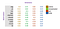Word-Vectors with undefined Dimensions

Similarity between word vectors is measured using Cosine similarity.

Nearest neighbors

The Euclidean distance (or cosine similarity) between two word vectors provides an effective method for measuring the linguistic or semantic similarity of the corresponding words. Sometimes, the nearest neighbors according to this metric reveal rare but relevant words that lie outside an average human’s vocabulary.

Skip-gram and CBOW

skip-gram takes the target/given word as the input and predicts the context words (in the window), whereas CBOW takes the context terms as the input and predicts the target/given term.

Simply put, the CBOW model learns the embedding by predicting the current word based on its context. The skip-gram model learns by predicting the surrounding words given a current word.

word embeddings trained using skip-grams are slightly ‘better’ than those trained using CBOW for less frequent words . By ‘better’, we simply mean that words similar to an infrequent word, will also be infrequent words.

Glove Embeddings

We had mentioned earlier that apart from Word2Vec, several other word embeddings have been developed by various teams. One of the most popular is GloVe (Global Vectors for Words) developed by a Stanford research group. These embeddings are trained on about 6 billion unique tokens and are available as pre-trained word vectors ready to use for text applications.

Probabilistic Latent Semantics Analysis (PLSA)

PLSA is a probabilistic technique for topic modelling. First, we fix an arbitrary number of topics which is a hyperparameter (for e.g.10 topics in all documents). The basic model we assume is this — each document is a collection of some topics and each topic is a collection of some terms.

The task of the PLSA algorithm is to figure out the set of topics c. PLSA is often represented as a graphical model with shaded nodes representing observed random variables (d, w) and unshaded ones unobserved random variables ©. The basic idea for setting up the optimization routine is to find the set of topics c which maximizes the joint probability P(d, w).

# Unsupervised Learning — Topic Modelling:: why do we even require it?

let me answer by supposing you are a product manager at Amazon and want to understand what features of a recently released product (say Amazon Echo Dot) customers are talking about in their reviews.

lets assume you are able to identify that 50% people talk about the hardware, 30% talk about features related to music, while 20% talk about the packaging of the product.

one another scenario could be where we have large corpus of some Research Papers and we want to build an application which does topic-specific search will make that all that easier.

what is Topic — Main Idea described by the Text

High Level Intuition behind Topics: let say a teacher delivering lecture about technology each day to a class of students.

I am one of his student transcribed all his Gyan and got few Keywords as below-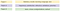Guess what the Topic is about!!

Topic Distribution:: (o.5, o.5, 0) :: ‘This is core Idea of Topic Modelling’.

The input to a topic model is the corpus of documents, for e.g. a set of customer reviews, tweets, research papers, books etc.

There are two outputs of a topic model — 1. The distribution of topics in a document and 2. the distribution of words in a topic.

likewise there are two Tasks in Topic Modelling —

1. Defining a Topic — easy, each Term is Topic — Distribution over a Vocabulary
2. Estimating Coverage

suppose we have two Topics Magic and Science —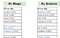Distribution over a Vocabulary

Probabilistic Latent Semantic Analysis (PLSA)

PLSA can be represented as a graphical model having the random variables documents d, topics c and words w.

The basic idea used to infer the parameters, i.e. the optimisation objective, is to maximise the joint probability p(w, d) of observing the documents and the words (since those two are the only observed variables). Notice that you are doing something very clever (and difficult) here — using the observed random variables (d, w) to infer the unobserved random variable c.

Using the Bayes’ rule, you can write p(w, d) as:

p(w,d) = p(d) x p(w|d)

“inference task is to figure out the M x k document-topic probabilities and the k x N topic-term probabilities.”

lets say there are M documents (represented by the outer plate in the figure below), and for simplicity, assume that there are N words in each document (the inner plate).

The term p(w|d) represents the probability of a word w being generated from a document d. But our model assumes that words are generated from topics, which in turn are generated from documents, so we can write p(w|d) as p(w|c). p(c|d) summed over all k topics:

P(w|d) = ∑p(c|d) x p(w|c)

So, we have P(w,d) = p(d) x ∑ [p(c|d) x p(w|c)]

Limitation of PLSA::main problem with PLSA is that it has a large number of parameters which grow linearly with the documents.”

# Latent Dirichlet Allocation (LDA) —

in LDA, we assume that the document-topic and topic-term distributions are Dirichlet distributions (parameterized by some variables), and we want to infer these two distributions.

below we have LDA Plate Diagram-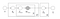LDA Plate

“Unlike PLSA, LDA is a parametric model, i.e. we dont have to learn all the individual probabilities. Rather, we assume that the probabilities come from an underlying probability distribution (the ‘Dirichlet’ distribution) which we can model using some parameters.”

for example in Normal Distribution we have two Parameters i.e Mean and Standard Deviation.in LDA, we assume that the document-topic and topic-term distributions are Dirichlet distributions (parameterized by some variables), and we want to infer these two distributions.

as referred to above Plate Diagram;

Alpha — “parameter of the Dirichlet distribution which determines the document-topic distribution.”
Eta — “parameter which determines the topic-term distribution.

# Dirichlet Distribution: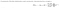Symmetric Dirichlet Distribution

At values of alpha < 1 (figure-4), most points are dispersed towards the edges apart from a few which are at the centre (a sparse distribution — most topics have low probabilities while a few are dominant).

At alpha=1 (figure-1) the points are distributed uniformly across the simplex.

At alpha > 1 (figure 2, top-right) the points are distributed around the centre (i.e. all topics have comparable probabilities such as (t1=0.32,t2=0.33,t3=0.35)).

The figure on bottom-left shows an asymmetric distribution (not used in LDA) with most points being close to topic-2.

Key Inferences::

Sparse Topics — very low alpha means that there is only one topic in the document or only one term in a given Topic — .
— if alpha is high, you are not even listening to the data but if its moderate, you have mix of given data and estimation — .

# Work in progress

As a Use Case I am working on scraping Tweets real time using Twitter Api and applying all the NLP techniques to create LDA models,word to vec,and extracting Narratives and clustering them.

Keep watching this workspace for more updates….

## More from Ashish Pandey

Email-pandeyrobin2609@gmail.com